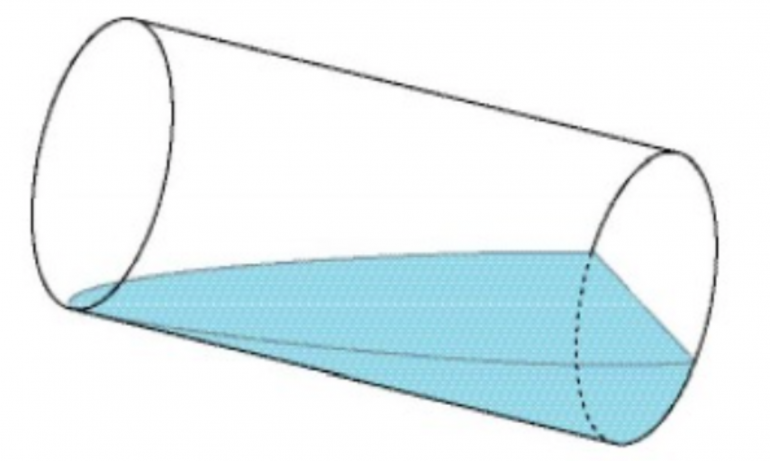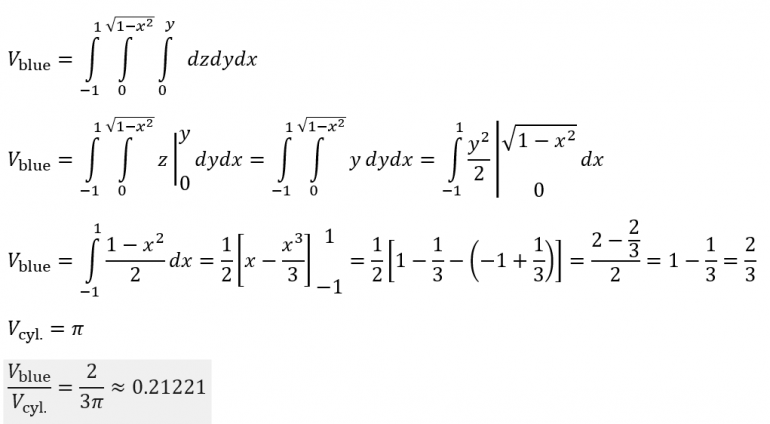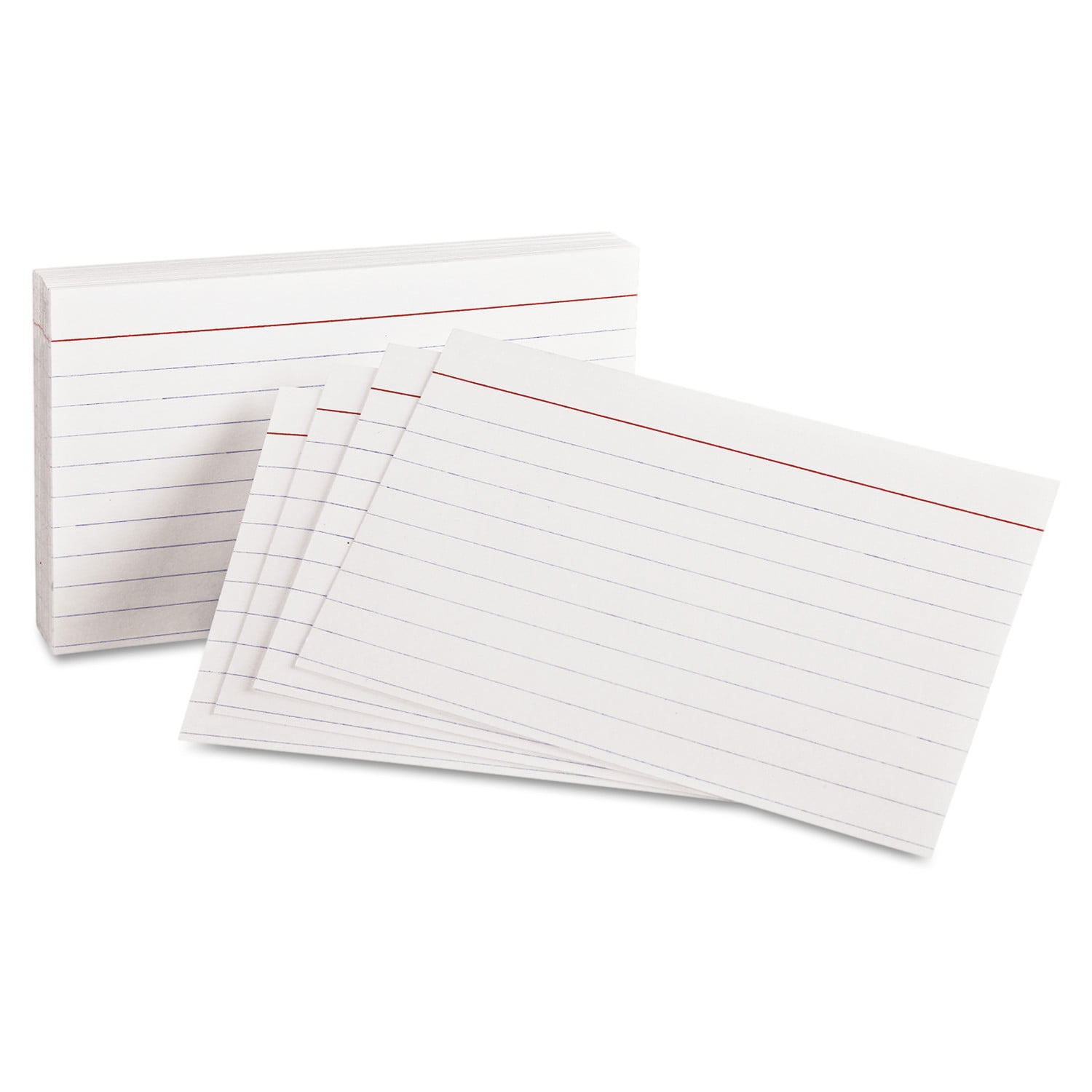## Poll

 I love math!20 votes (46.51%) Math is great.14 votes (32.55%) My religion is mathology.6 votes (13.95%) Women didn't speak to me until I was 30.2 votes (4.65%) Total eclipse reminder -- 04/08/202412 votes (27.9%) I steal cutlery from restaurants.3 votes (6.97%) I should just say what's on my mind.6 votes (13.95%) Who makes up these awful names for pandas?5 votes (11.62%) I like to touch my face.12 votes (27.9%) Pork chops and apple sauce.9 votes (20.93%)

43 members have voted

ThatDonGuyJoined: Jun 22, 2011
• Posts: 5680
Thanks for this post from:June 16th, 2021 at 8:38:30 AM permalink
Quote: aceside

Can you also find some successive consecutive cubes for fun? Just replace all of the second power to the third power.

I'll see what I can do.

Cubes should be easier, as 1^3 + 2^3 + ... + n^3 = (1 + 2 + ... + n)^2
(n-(a-1))^3 + (n-(a-2))^3 + ... + n^3 = (n+1)^3 + (n+2)^3 + ... + (n+b)^3
you get:
(n (n + 1) / 2)^2 - ((n - a) (n - a + 1) / 2)^2 = ((n + b)(n + b + 1) / 2)^2 = (n (n + 1) / 2)^2
2 (n (n + 1))^2 = ((n + b) (n + b + 1))^2 + ((n-a) (n - a + 1))^2

So far, the only one I have found is 3^3 + 4^3 + 5^3 = 6^3 = 216
GialmereJoined: Nov 26, 2018
• Posts: 2589
June 16th, 2021 at 8:44:35 AM permalinkFill a cylindrical glass with water. A quick way to empty it to exactly half its volume is to pour out the water until the water line just touches the circular bottom of the glass. Suppose you tip the glass further, until the water line crosses the center of the bottom of the glass (forming a diameter of the bottom circle).

What fraction of the glass' volume is now filled with water?
Have you tried 22 tonight? I said 22.
teliotJoined: Oct 19, 2009
• Posts: 2496
June 16th, 2021 at 9:46:15 AM permalink
Quote: ThatDonGuy

I'll see what I can do.

Cubes should be easier, as 1^3 + 2^3 + ... + n^3 = (1 + 2 + ... + n)^2
(n-(a-1))^3 + (n-(a-2))^3 + ... + n^3 = (n+1)^3 + (n+2)^3 + ... + (n+b)^3
you get:
(n (n + 1) / 2)^2 - ((n - a) (n - a + 1) / 2)^2 = ((n + b)(n + b + 1) / 2)^2 = (n (n + 1) / 2)^2
2 (n (n + 1))^2 = ((n + b) (n + b + 1))^2 + ((n-a) (n - a + 1))^2

So far, the only one I have found is 3^3 + 4^3 + 5^3 = 6^3 = 216

Within the range of the INT data size that's the only one I found as well.
Climate Casino: https://climatecasino.net/climate-casino/
ThatDonGuyJoined: Jun 22, 2011
• Posts: 5680
June 16th, 2021 at 9:46:43 AM permalink
Quote: Ace2

Incidentally, my method uses the same concept as the Wizard's but it's not exactly the same. I took 1 minus the integral over all time of:

(1-((1-1/e^(t/210))*(1-1/e^(t/60))*(1-1/e^(t/30))*(1-1/e^(4t/75))*(1-1/e^(5t/66)))^2)*1/e^(7303t/11550)*7303/11550 dt

Let me see if I have this straight:
1 - 1/e^(t/210) is the probability that at least one point of 2 will be rolled and then made in time t (probability = 1/30 that a 2 will be rolled (ignoring 7s on the comeout) x 1/7 that a 2 will be rolled again before a 7 = 1/210)
1 - 1/e^(t/60) is the probability that at least one point of 3 will be rolled and then made in time t
1 - 1/e^(t/30) is the probability that at least one point of 4 will be rolled and then made in time t
...
1 - 1/e^(t/60) is the probability that at least one point of 11 will be rolled and then made in time t
1 - 1/e^(t/210) is the probability that at least one point of 12 will be rolled and then made in time t
The product is the probabiity that all 10 points will be rolled and then made in time t, so 1 - the product is the probability that at least one point will not be rolled and then made
1/e^(t*7303/11550) is the probability that a point will be rolled and then missed (i.e. a seven-out), so multiply this by the previous product to get the probability of sevening out before making all ten points
But why do you then multiply by 7303/11550 (the probability of sevening out in a particular pass)?
ThatDonGuyJoined: Jun 22, 2011
• Posts: 5680
June 16th, 2021 at 9:48:10 AM permalink
Quote: teliot

Within the range of the INT data size that's the only one I found as well.

I got as far as N = 13,000 (N is the largest term on the left side), and also could not find any others.
ChesterDogJoined: Jul 26, 2010
• Posts: 1136
Thanks for this post from:June 16th, 2021 at 9:54:45 AM permalink
Quote: GialmereFill a cylindrical glass with water. A quick way to empty it to exactly half its volume is to pour out the water until the water line just touches the circular bottom of the glass. Suppose you tip the glass further, until the water line crosses the center of the bottom of the glass (forming a diameter of the bottom circle).

What fraction of the glass' volume is now filled with water?

Let the height and radius of the glass equal 1. Then:Ace2Joined: Oct 2, 2017
• Posts: 2318
June 16th, 2021 at 10:18:39 AM permalink
Quote: ThatDonGuy

Let me see if I have this straight:
1 - 1/e^(t/210) is the probability that at least one point of 2 will be rolled and then made in time t (probability = 1/30 that a 2 will be rolled (ignoring 7s on the comeout) x 1/7 that a 2 will be rolled again before a 7 = 1/210)
1 - 1/e^(t/60) is the probability that at least one point of 3 will be rolled and then made in time t
1 - 1/e^(t/30) is the probability that at least one point of 4 will be rolled and then made in time t
...
1 - 1/e^(t/60) is the probability that at least one point of 11 will be rolled and then made in time t
1 - 1/e^(t/210) is the probability that at least one point of 12 will be rolled and then made in time t
The product is the probabiity that all 10 points will be rolled and then made in time t, so 1 - the product is the probability that at least one point will not be rolled and then made
1/e^(t*7303/11550) is the probability that a point will be rolled and then missed (i.e. a seven-out), so multiply this by the previous product to get the probability of sevening out before making all ten points
But why do you then multiply by 7303/11550 (the probability of sevening out in a particular pass)?

You have it mostly correct. Ignoring 7s on come out rolls, there are 11 possibilities: 1/210 winning a 2, 1/210 of winning a 12, 1/60 if winning a 3...etc and a 7303/11550 chance of sevening-out. As mentioned, these probabilities add up to 1.

The second to last term of 1/e^(7303t/11550) is the probability that zero seven-outs have happened. And, as you know, the previous terms represent the probability that at least 1 of the 10 points has not been won. Therefore, starting from this state, if the next decision is a seven-out then the fire bet loses at that point in time t. There is a 7303/11550 chance of that happening so we multiply by that factor. Combining all that and integrating gives the sum of the probabilities, at all times from zero to infinity, that the fire bet will lose at that point in time.
It�s all about making that GTA
ThatDonGuyJoined: Jun 22, 2011
• Posts: 5680
June 16th, 2021 at 10:28:24 AM permalink
Quote: Ace2

You have it mostly correct. Ignoring 7s on come out rolls, there are 11 possibilities: 1/210 winning a 2, 1/210 of winning a 12, 1/60 if winning a 3...etc and a 7303/11550 chance of sevening-out. As mentioned, these probabilities add up to 1.

The second to last term of 1/e^(7303t/11550) is the probability that zero seven-outs have happened. And, as you know, the previous terms represent the probability that at least 1 of the 10 points has not been won. Therefore, starting from this state, if the next decision is a seven-out then the fire bet loses at that point in time t. There is a 7303/11550 chance of that happening so we multiply by that factor. Combining all that and integrating gives the sum of the probabilities, at all times from zero to infinity, that the fire bet will lose at that point in time.

So the last part of my explanation should be:
1/e^(t*7303/11550) is the probability that a point will not be rolled and then missed (i.e. a seven-out), so multiply this by the previous product to get the probability of not rolling and making every point nor missing any points (i.e. the bet is "still active").
Multiply this by 7303/11550 to get the probability of sevening out before making all ten points.
Subtract this result from 1 to get the probability of making all ten points before sevening out (i.e. winning the fire bet).
GialmereJoined: Nov 26, 2018
• Posts: 2589
June 16th, 2021 at 4:34:21 PM permalink
Quote: ChesterDog

Let the height and radius of the glass equal 1. Then:Correct!!

lol! Apologies for the size of that picture. I was trying to whip out a puzzle on my cell at work. Heh, it looks just fine on a phone screen.
--------------------------------------------------------------

An optimist sees the glass as half full.

A pessimist sees the glass as half empty.

An engineer sees the glass as a waste of space and money since it's twice as big as you need.
Have you tried 22 tonight? I said 22.
GialmereJoined: Nov 26, 2018
• Posts: 2589
June 17th, 2021 at 8:01:51 AM permalink1) Oh no! You want to play a board game but the box is missing its pair of standard dice. You look around and see some 3x5 cards. Hmm. Woo hoo! You can make two piles of six cards, each numbered 1-6, and Bam! By randomly drawing one card from each pile you have a "pair of dice".

D'oh! There's only eight cards. Hmm. A ha! You can still divide the cards into two sets (piles) and number them in such a way that by drawing two cards from each pile, and adding the four cards together, you get an integer from 2 to 12 with the same frequency of occurrence as rolling that sum on two standard dice.

How do you do it?

2) But wait! You recount and find there are actually nine cards available. Hmm. It occurs to you that you could number the nine cards in such a way that randomly drawing two cards from the pile of nine cards also gives an integer from 2 to 12 with the same frequency of occurrence as rolling that sum on two standard dice.

How do you do it?Have you tried 22 tonight? I said 22.